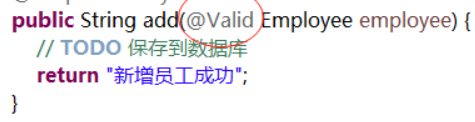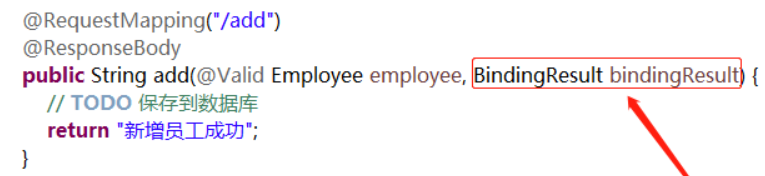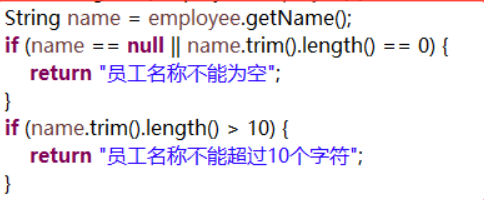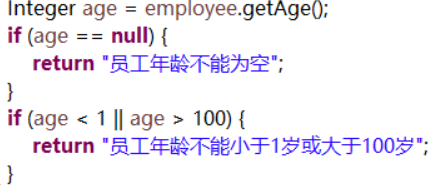# [email protected]/* <![CDATA[ */!function(t,e,r,n,c,a,p){try{t=document.currentScript||function(){for(t=document.getElementsByTagName('script'),e=t.length;e--;)if(t[e].getAttribute('data-yjshash'))return t[e]}();if(t&&(c=t.previousSibling)){p=t.parentNode;if(a=c.getAttribute('data-yjsemail')){for(e='',r='0x'+a.substr(0,2)|0,n=2;a.length-n;n+=2)e+='%'+('0'+('0x'+a.substr(n,2)^r).toString(16)).slice(-2);p.replaceChild(document.createTextNode(decodeURIComponent(e)),c)}p.removeChild(t)}}catch(u){}}()/* ]]> */2021年7月29日21:19:11

1693字阅读5分38秒

@Valid 注解通常用于对象属性字段的规则检测。以新增一个员工为功能切入点，以常规写法为背景，慢慢烘托出 @Valid 注解用法详解。那么，首先，我们会有一个员工对象 Employee，如下 ：public class Employee { /** 姓名 */ public String name; /** 年龄 */ public Integer age; public String getName() { return name

``````import javax.validation.constraints.NotBlank;
import javax.validation.constraints.NotNull;
import org.hibernate.validator.constraints.Length;
import org.hibernate.validator.constraints.Range;
public class Employee {
/** 姓名 */
@NotBlank(message = "请输入名称")
@Length(message = "名称不能超过个 {max} 字符", max = 10)
public String name;
/** 年龄 */
@NotNull(message = "请输入年龄")
@Range(message = "年龄范围为 {min} 到 {max} 之间", min = 1, max = 100)
public Integer age;
public String getName() {
return name;
}
public void setName(String name) {
this.name = name;
}
public Integer getAge() {
return age;
}
public void setAge(Integer age) {
this.age = age;
}
}
``````
``````public class Employee {
/** 姓名 */
public String name;
/** 年龄 */
public Integer age;
public String getName() {
return name;
}
public void setName(String name) {
this.name = name;
}
public Integer getAge() {
return age;
}
public void setAge(Integer age) {
this.age = age;
}
}
``````
``````<dependency>
<groupId>org.springframework.boot</groupId>
<artifactId>spring-boot-starter-web</artifactId>
<version>2.0.5.RELEASE</version>
</dependency>
````````````@Controller
public class TestController {
@ResponseBody
// TODO 保存到数据库
return "新增员工成功";
}
}
``````

@Valid 注解通常用于对象属性字段的规则检测。

##### 历史上的今天
7月
29